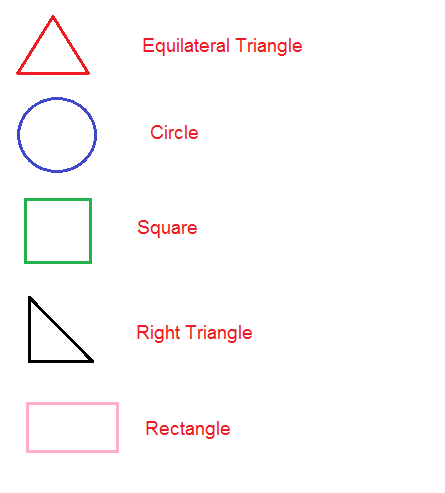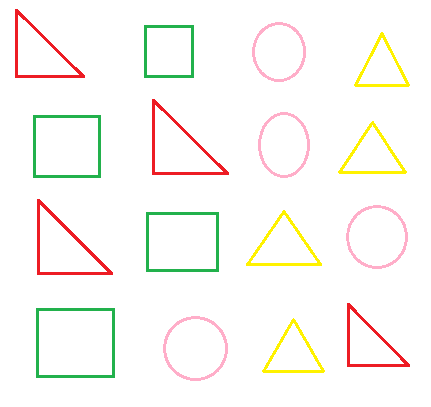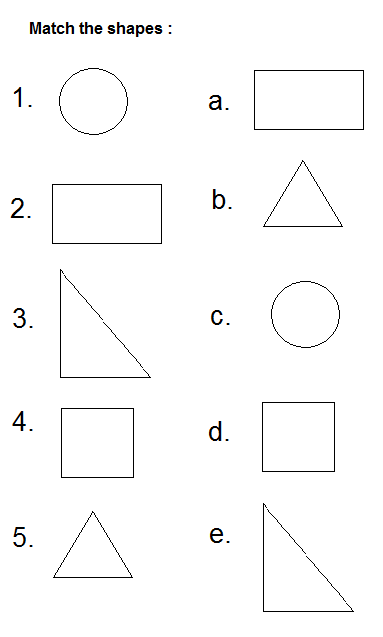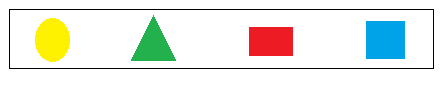## Geometry Worksheets

### First Grade Geometry worksheets :Answer the question from above Geometry Figures:

1 . How many circles are there in this figure?

2. How many right triangle in this figure?Count and color :

Count and color the correct number of each shape in the box below :